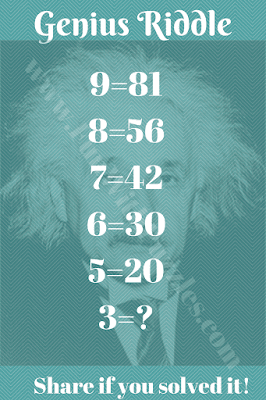Can you solve this Genius Riddle?Can you solve this Genius Riddle?

#### 4 comments:

1.The answer is 6 bcse.
3×3=9-3=6
Multiply on it's self then, substruct on the original number I mean it's self.😉

1.Yes, your answer to this genius riddle is correct.

2.Then, that makes the first equation invalid.

3.If the answeris 6 then the first equation is invalid.

It will be great to read about your comments about this post.